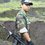# Open Problem (Calculus - 1)

Evaluate the following improper integrals and sum of series:

$\Large\color{blue}{\int_{-\infty}^\infty\frac{x\sin\alpha x}{x^4+x^2+1}\ dx}$

$\Large\color{#D61F06}{\int_{-\infty}^\infty\frac{\cos\beta x}{x^6+1}\ dx}$

$\Large\color{#456461}{\sum_{n=-\infty}^\infty\frac{(-1)^n}{n^2+\gamma^2}}$Note by Tunk-Fey Ariawan
6 years, 10 months ago

This discussion board is a place to discuss our Daily Challenges and the math and science related to those challenges. Explanations are more than just a solution — they should explain the steps and thinking strategies that you used to obtain the solution. Comments should further the discussion of math and science.

When posting on Brilliant:

• Use the emojis to react to an explanation, whether you're congratulating a job well done , or just really confused .
• Ask specific questions about the challenge or the steps in somebody's explanation. Well-posed questions can add a lot to the discussion, but posting "I don't understand!" doesn't help anyone.
• Try to contribute something new to the discussion, whether it is an extension, generalization or other idea related to the challenge.
• Stay on topic — we're all here to learn more about math and science, not to hear about your favorite get-rich-quick scheme or current world events.

MarkdownAppears as
*italics* or _italics_ italics
**bold** or __bold__ bold
- bulleted- list
• bulleted
• list
1. numbered2. list
1. numbered
2. list
Note: you must add a full line of space before and after lists for them to show up correctly
paragraph 1paragraph 2

paragraph 1

paragraph 2

[example link](https://brilliant.org)example link
> This is a quote
This is a quote
    # I indented these lines
# 4 spaces, and now they show
# up as a code block.

print "hello world"
# I indented these lines
# 4 spaces, and now they show
# up as a code block.

print "hello world"
MathAppears as
Remember to wrap math in $$ ... $$ or $ ... $ to ensure proper formatting.
2 \times 3 $2 \times 3$
2^{34} $2^{34}$
a_{i-1} $a_{i-1}$
\frac{2}{3} $\frac{2}{3}$
\sqrt{2} $\sqrt{2}$
\sum_{i=1}^3 $\sum_{i=1}^3$
\sin \theta $\sin \theta$
\boxed{123} $\boxed{123}$

## Comments

Sort by:

Top Newest

The third one is equal to $\frac{\pi}{\gamma} \text{csch}\left(\gamma\pi\right)$, where csch is the hyperbolic cosecant. The first step is to take $\frac{(-1)^n}{n^2+\gamma^2} = \sum_{k=0}^{\infty} \frac{(-1)^{n+k} \gamma^{2k}}{n^{2k+2}}$ We can then switch the order to $\lim_{a\to\infty}\,\sum_{k=0}^{\infty} \sum_{n=-a}^{a} \frac{(-1)^{n+k} \gamma^{2k}}{n^{2k+2}}$ to get $\sum_{k=0}^{\infty} (-1)^{k-1} \left(2-4^{-k}\right) \zeta (2 k+2)\gamma^{2k}$ This of course neglects the $\frac{(-1)^0}{0^2+\gamma^2}$ term (which is undefined for $n = 0$ in the denominator of the summand), so we must add $\frac{1}{\gamma^2}$ to our previous sum to get $\frac{1}{\gamma^2} + \sum_{k=0}^{\infty} (-1)^{k-1} \left(2-4^{-k}\right) \zeta (2 k+2)\gamma^{2k}$ We can see the relation between these coefficients and the coefficients of the series for the hyperbolic cosecant to get that our answer should be $\boxed{\frac{\pi}{\gamma} \text{csch}\left(\gamma\pi\right)}$.

- 6 years, 10 months ago

Log in to reply

Excellent!! How about this one. $\ddot\smile$

- 6 years, 10 months ago

Log in to reply

Lol this is what I keep doing too - directing people to the IMPOSSIBRU integral xD

- 6 years, 9 months ago

Log in to reply

Actually, I got the correct answer to that one by hand-estimating. I'm fairly certain that your answer is not exactly correct. To 50 digits, the integral is 0.59738180945180348461311323509087376430643859042556, whereas to 50 digits, the right side is 0.59738519747762744103977711867133271207190293243263.

- 6 years, 10 months ago

Log in to reply

minyak

- 6 years, 5 months ago

Log in to reply

Quick sketch : the first two can be done using complex analysis just consider the integral over a half circle from $R$ to $-R$ counterclockwise and $(-R,R)$.

For the sum, consider the integral of $z\mapsto \frac{\pi \csc(\pi z)}{z^2+x^2}$ over a rectangle with vertices $(n+1/2)\left\{1+i,1-i,-1+i,-1-i\right\}$.

I can't write full solutions now, but I'll write when have time.

- 6 years, 10 months ago

Log in to reply

+1 for your comment. I know how to evaluate them using residue method but I am interested in evaluating them using elementary methods. Anyway, could you please post your solution to this problem: OMG !! Zeta and Pi in one integral!. Thank you.

- 6 years, 10 months ago

Log in to reply

@Tunk-Fey Ariawan Sorry, I do not have much time and the evaluation of $\psi_4(1/3)$ is lengthy. To simplify Pranav's result you have only to calculate $\psi_4(1/3)$ (using some basic polygamma identities).

- 6 years, 10 months ago

Log in to reply

I did solve your problem in similar fashion as @Pranav Arora did. I thought you had a different approach since you commented to him: "Have you noticed that the fraction has a partial fraction decomposition that might help?"

- 6 years, 10 months ago

Log in to reply

@Tunk-Fey Ariawan I've added a solution to that problem. you may see that it is long, that's why I couldn't post it until I had time.

- 6 years, 10 months ago

Log in to reply

It look like we did it by using the same approach. Nice solution!

- 6 years, 10 months ago

Log in to reply

Problem No 2

Answer is $\displaystyle \boxed{\frac{\pi e^{-\beta}}{6} + \frac{\pi}{\sqrt3}e^{-\beta/2}\left(\sin(\frac{\sqrt3 \beta}{2})+\cos(\frac{\sqrt3 \beta}{2})\right)}$

- 5 years, 3 months ago

Log in to reply

please anybody solve the integral using the residue theorem

- 6 years, 9 months ago

Log in to reply

Problem No 1

Answer is

$\displaystyle \boxed{\frac{2\pi}{\sqrt3}e^{-\frac{\sqrt3 \alpha}{2}}\sin(\frac{\alpha}{2})}$

- 5 years, 3 months ago

Log in to reply

×

Problem Loading...

Note Loading...

Set Loading...